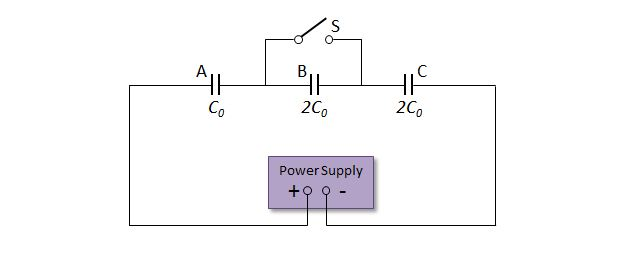# Circuit with three capacitors 2The above diagram is a circuit that consists of three capacitors A, B and C with capacitances $C_0,$ $2C_0$ and $2C_0,$ respectively. The voltage of the power supply is constant. Let $E_1$ be the electric energy charged on capacitor A when the switch S is open. Let $E_2$ be the electric energy charged on capacitor A when the switch S is closed. Then what is the ratio $E_1:E_2?$

×